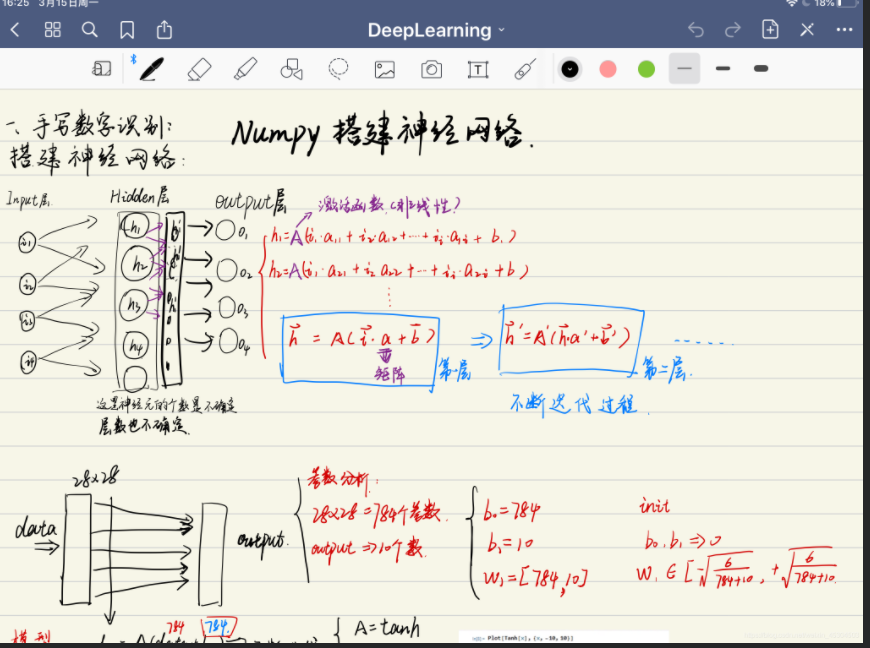# 基于numpy的前馈神经网络(feedforward neural network)

+关注继续查看

***

## 前馈神经网络$z^{(i)} = W^{} x^{(i)} + b^{}$
$a^{(i)} = \tanh(z^{(i)})$
$z^{(i)} = W^{} a^{(i)} + b^{}$
$\hat{y}^{(i)} = a^{(i)} = \sigma(z^{ (i)})$
$y^{(i)}_{prediction} = \begin{cases} 1 & \mbox{if } a^{(i)} > 0.5 \\ 0 & \mbox{otherwise } \end{cases}$

$sigmoid(z) = \frac{1}{1+e^{-z}}$
$tanh(z) = \frac{e^{z} - e^{-z}}{e^{z} + e^{-z}} = 2 sigmoid(2z) - 1$
$relu(z) = max(0, z) = begin{cases} z, & z>0 \\ 0, & z leq 0 end{cases}$$J = -\frac{1}{m} \sum_{i = 1}^{m}{ [ y^{(i)}log(\hat{y}^{(i)}) + (1-y^{(i)}) log(1-\hat{y}^{(i)}) ] }$

$Z^{} = W^{}X + b^{}$
$A^{} = g^{}(Z^{})$
$Z^{} = W^{}X + b^{}$
$A^{} = g^{}(Z^{})$
$...$
$A^{[L]} = g^{[L]}(Z^{[L]}) = \hat{Y}$

$W^{[l]} = W^{[l]} - \alpha \text{ } dW^{[l]}$
$b^{[l]} = b^{[l]} - \alpha \text{ } db^{[l]}$
$\alpha:learning \text{ }rate$
$dW \text{ | } db: gradients$• 确定神经网络的层数和每层的神经元数
• 初始化参数W和b
• 进行循环迭代

• 前向传播，得到预测值
• 计算损失函数
• 反向传播，得到参数W和b的梯度
• 对参数W和b进行更新
• 对新数据做预测

## 代码实现def sigmoid(z):
'''
z为prev_activation, size为 nl * m
'''
return 1 / (1 + np.exp(-z))

def relu(z):
'''
z为prev_activation, size为 nl * m
'''
return np.maximum(0, z)

n0, m = X.shape
n1 = 20
n2 = 7
n3 = 5
n4 = 1
layers_dims = [n0, n1, n2, n3, n4] # [12288, 20, 7, 5, 1]
L = len(layers_dims) - 1  # 4层神经网络，不计输入层

##### neural network model
def neural_network(X, Y, learning_rate=0.01, num_iterations=2000, lambd=0):
m = X.shape
### initialize forward propagation
param_w = [i for i in range(L+1)]
param_b = [i for i in range(L+1)]
np.random.seed(10)
for l in range(1, L+1):
if l < L:
param_w[l] = np.random.randn(layers_dims[l], layers_dims[l - 1]) * np.sqrt(2 / layers_dims[l - 1])
if l == L:
param_w[l] = np.random.randn(layers_dims[l], layers_dims[l - 1]) * 0.01
param_b[l] = np.zeros((layers_dims[l], 1))

activations = [X, ] + [i for i in range(L)]
prev_activations = [i for i in range(L+1)]

dA = [i for i in range(L+1)]
dz = [i for i in range(L+1)]
dw = [i for i in range(L+1)]
db = [i for i in range(L+1)]

for i in range(num_iterations):
### forward propagation
for l in range(1, L+1):
prev_activations[l] = np.dot(param_w[l], activations[l-1]) + param_b[l]
if l < L:
activations[l] = relu(prev_activations[l])
else:
activations[l] = sigmoid(prev_activations[l])

cross_entropy_cost = -1/m * (np.dot(np.log(activations[L]), Y.T) \
+ np.dot(np.log(1-activations[L]), 1-Y.T))
regularization_cost = 0
for l in range(1, L+1):
regularization_cost += np.sum(np.square(param_w[l])) * lambd/(2*m)
cost = cross_entropy_cost + regularization_cost

### initialize backward propagation
dA[L] =  np.divide(1-Y, 1-activations[L]) - np.divide(Y, activations[L])
assert dA[L].shape == (1, m)

### backward propagation
for l in reversed(range(1, L+1)):
if l == L:
dz[l] = dA[l] * activations[l] * (1-activations[l])
else:
dz[l] = dA[l].copy()
dz[l][prev_activations[l] <= 0] = 0

dw[l] = 1/m * np.dot(dz[l], activations[l-1].T) + param_w[l] * lambd/m
db[l] = 1/m * np.sum(dz[l], axis=1, keepdims=True)
dA[l-1] = np.dot(param_w[l].T, dz[l])

assert dz[l].shape == prev_activations[l].shape
assert dw[l].shape == param_w[l].shape
assert db[l].shape == param_b[l].shape
assert dA[l-1].shape == activations[l-1].shape

param_w[l] = param_w[l] - learning_rate * dw[l]
param_b[l] = param_b[l] - learning_rate * db[l]

if i % 100 == 0:
print("cost after iteration {}: {}".format(i, cost))

Andrew Ng教授是用一个dict来保存每层神经元的参数的，比如说，在调用第三层的参数W3和b3时，他的写法是:parameters['W' + str(3)]parameters['b' + str(3)]，这样写没有错误，虽然直观，但是很麻烦，我的做法是，分别使用list来保存W和b，根据位置读取，对应上面的就是param_wparam_b

for l in reversed(range(L - 1)):
# lth layer: (RELU -> LINEAR) gradients.
# Inputs: "grads["dA" + str(l + 1)], current_cache".
# Outputs: "grads["dA" + str(l)] , grads["dW" + str(l + 1)] , grads["db" + str(l + 1)]

### START CODE HERE ### (approx. 5 lines)
current_cache = caches[l]
dA_prev_temp, dW_temp, db_temp = linear_activation_backward(grads['dA' + str(l + 1)], current_cache, activation="relu")
grads["dW" + str(l + 1)] = dW_temp
grads["db" + str(l + 1)] = db_temp
### END CODE HERE ###

Ng教授的代码中，对于不同的子函数，层数L时而等于5，时而等于4，很是混乱，而我的层数L始终等于4，符合python从0开始计数的习惯，使得整个算法写起来很快，也很好理解，一看就明白当前迭代到了哪一层。def predict(X_new, parameters, threshold=0.5):
param_w = parameters["param_w"]
param_b = parameters["param_b"]

activations = [X_new, ] + [i for i in range(L)]
prev_activations = [i for i in range(L + 1)]
m = X_new.shape

for l in range(1, L + 1):
prev_activations[l] = np.dot(param_w[l], activations[l - 1]) + param_b[l]
if l < L:
activations[l] = relu(prev_activations[l])
else:
activations[l] = sigmoid(prev_activations[l])
prediction = (activations[L] > threshold).astype("int")
return prediction

## 经验与收获

• 这是我第一次用python写一个具体的算法，虽然一直在python做数据分析，但是一直没有写过一个逻辑完整的、能应用于实际场合的算法（自己动手写完整算法的经历，在今天之前，是用R语言写了逐步回归和随机森林），今天算是填补了我在python上的这块空白。
• 使用assert语句来确保每个参数的shape正确，能够减少出现bug的几率
• numpy中有一些操作和函数是element-wise的，要留意
• 整个算法没一会儿就写完了，但是训练的时候cost一直没有收敛，检查了老半天，才发现是由两个问题导致的：

• relu的导函数写错了，返回值是0与1没错，但是我用来判断的参数竟然是A而不是z，真是写得莫名其妙；
• 初始化不正确（我后来才发现初始化是神经网络的关键），对于权重W我一直使用的写法是param_w[l] = np.random.randn(layers_dims[l], layers_dims[l - 1]) * 0.01，导致cost没能实现收敛，应该是由vanishing gradients导致的，后来我使用了He初始化和Xavier初始化，即把上面的0.01改成np.sqrt(2 / layers_dims[l - 1])或者np.sqrt(1 / layers_dims[l - 1])，效果明显。
• 怎么知道自己的最终算法写对了？我和课程使用的是同一套数据，如果使用我的算法，在相同的神经网络结构下，和Ng教授使用的算法得到的精确度差不多，就说明没问题了。
• 比起写代码，理解代码背后的算法内容更重要。|
8天前
|

Python 数据分析入门教程：Numpy、Pandas、Matplotlib和Scikit-Learn详解
Python 数据分析入门教程：Numpy、Pandas、Matplotlib和Scikit-Learn详解
26 0
|

​ 利用纯numpy实现手势识别，首先是进行的整体的网络构成，然后再展示代码部分。这是我的第一个神经网络。 完整代码： GitHub 网络大体体现： ​ 输入层，隐藏层，输出层。已经知道的是输出层是有十个结果的，就是10个数字的概率。
92 0|

2459 0
|